## Tamilnadu Samacheer Kalvi 9th Maths Solutions Chapter 7 Mensuration Ex 7.4

Multiple Choice Questions:

Question 1.
The semi-perimeter of a triangle having sides 15 cm, 20 cm and 25 cm is
(1) 60 cm
(2) 45 cm
(3) 30 cm
(4) 15 cm
Solution:
(3) 30 cm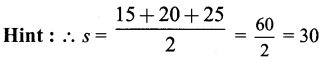Question 2.
If the sides of a triangle are 3 cm, 4 cm and 5 cm, then the area is
(1) 3 cm2
(2) 6 cm2
(3) 9 cm2
(4) 12 cm2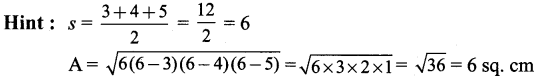Solution:
(2) 6 cm2

Question 3.
The perimeter of an equilateral triangle is 30 cm. The area is
(1) $$10 \sqrt{3}$$ cm2
(2) $$12 \sqrt{3}$$ cm2
(3) $$15 \sqrt{3}$$ cm2
(4) $$25 \sqrt{3}$$ cm2
Solution:
3a = 30
a = 10 cm

Solution:
(4) $$25 \sqrt{3}$$ cm2

Question 4.
The lateral surface area of a cube of side 12 cm is
(1) 144 cm2
(2) 196 cm2
(3) 576 cm2
(4) 664 cm2
Hint: 4a2 = 4 × 12 × 12 = 4 × 144 = 576 cm2
Solution:
(3) 576 cm2

Question 5.
If the lateral surface area of a cube is 600 cm2, then the total surface area is
(1) 150 cm2
(2) 400 cm2
(3) 900 cm2
(4) 1350 cm2
Hint:
4a2 = 600 cm2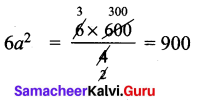Solution:
(3) 900 cm2Question 6.
The total surface area of a cuboid with dimension 10 cm × 6 cm × 5 cm is
(1) 280 cm2
(2) 300 cm2
(3) 360 cm2
(4) 600 cm2
Hint:
TSA = 2 (lb + bh + hl)
= 2 (10 × 6 + 6 × 5 + 5 × 10) = 2 (60 + 30 + 50) = 2 (140) = 280 cm2
Solution:
(1) 280 cm2

Question 7.
If the ratio of the sides of two cubes are 2 : 3, then ratio of their surface areas will be
(1) 4 : 6
(2) 4 : 9
(3) 6 : 9
(4) 16 : 36
Hint:
a : b = 2 : 3
4a2 : 4b2 = 4 × 22 : 4 : 32
⇒ 16 : 36
⇒ 4 : 9
Solution:
(2) 4 : 9

Question 8.
The volume of a cuboid is 660 cm3 and the area of the base is 33 cm2. Its height is
(1) 10 cm
(2) 12 cm
(3) 20 cm
(4) 22 cm
Hint:
V = 660 cm3
lb = 33 cm2
h = ?
lbh = 660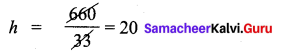Solution:
(3) 20 cm

Question 9.
The capacity of a water tank of dimensions 10 m × 5 m × 1.5 m is
(1) 75 litres
(2) 750 litres
(3) 7500 litres
(4) 75000 litres
Hint: Volume of cuboid = l × b × h
Solution:
(4) 75000 litresQuestion 10.
The number of bricks each measuring 50 cm × 30 cm × 20 cm that will be required to build a wall whose dimensions are 5m × 3m × 2m is
(1) 1000
(2) 2000
(3) 3000
(4) 5000
Hint: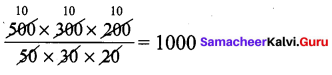Solution:
(1) 1000